# On a squared paper, sketch the following:(a) A triangle with a horizontal line of symmetry but no vertical line of symmetry.(b) A quadrilateral with both horizontal and vertical lines of symmetry.(c) A quadrilateral with a horizontal line of symmetry but no vertical line of symmetry.(d) A hexagon with exactly two lines of symmetry.(e) A hexagon with six lines of symmetry.(Hint : It will be helpful if you first draw the lines of symmetry and then complete the figures.)

To do:

We have to sketch the given figures on a squared paper.

Solution:

Line of Symmetry:

It is a line that divides the figure into two symmetrical parts in such a way that the figure on one side is the mirror image of the figure on the other side.

Therefore,

(a) A triangle with a horizontal line of symmetry but no vertical line of symmetry can be drawn as follows: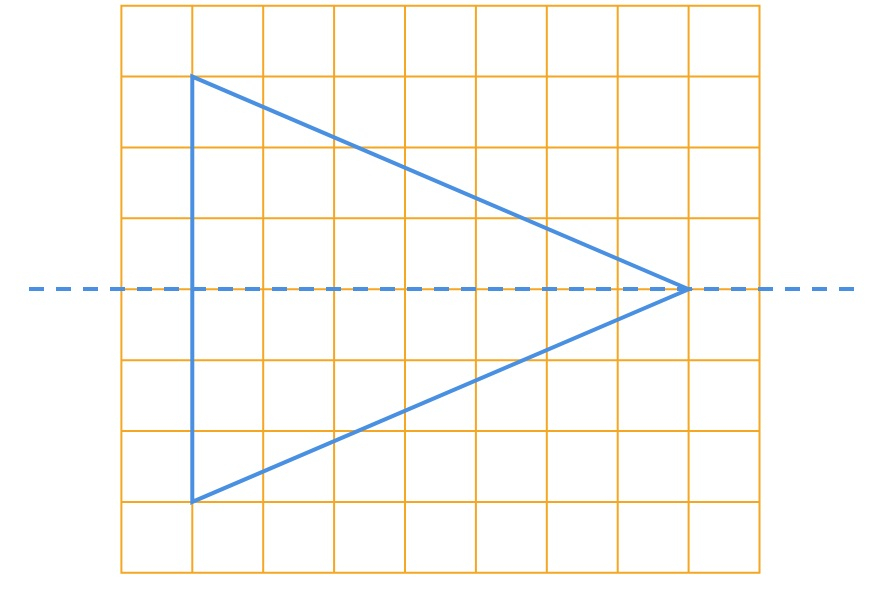(b) A quadrilateral with both horizontal and vertical lines of symmetry can be drawn as follows: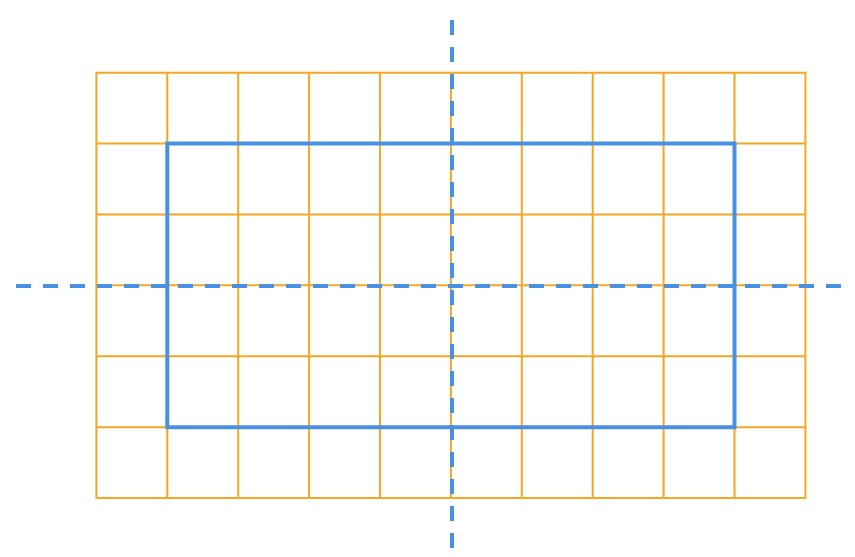(c) A quadrilateral with a horizontal line of symmetry but no vertical line of symmetry can be drawn as follows: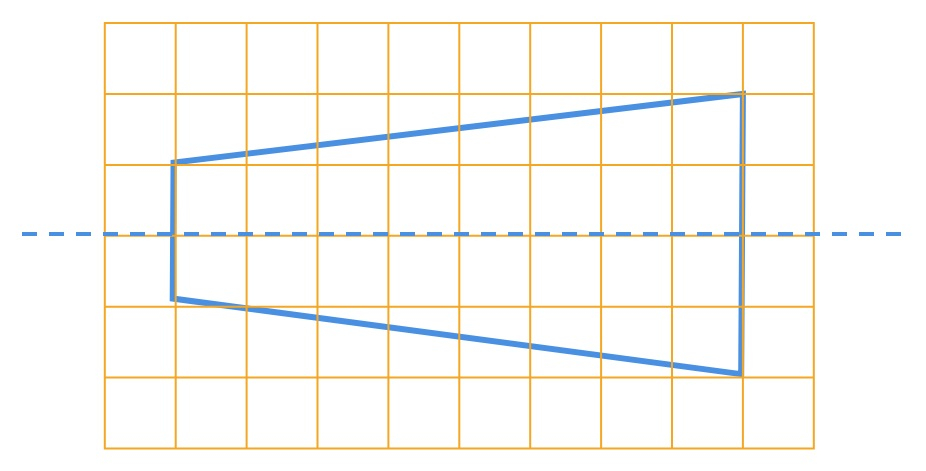(d) A hexagon with exactly two lines of symmetry can be drawn as follows: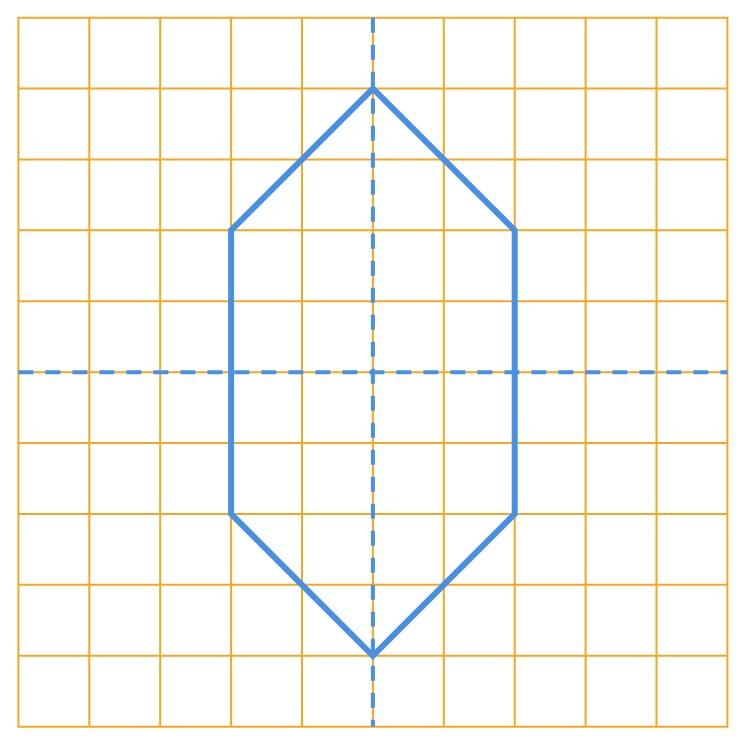(e) A hexagon with six lines of symmetry can be drawn as follows: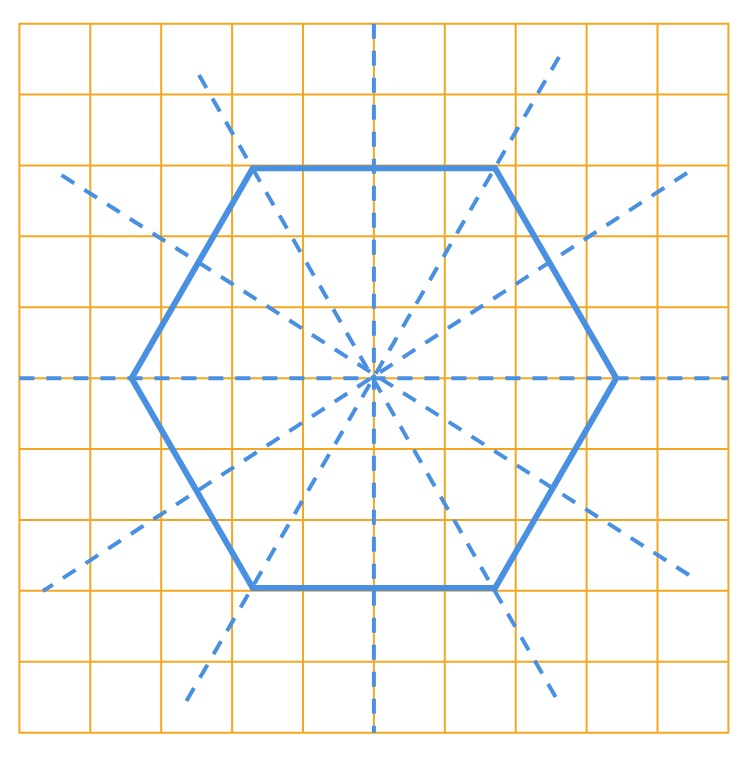Updated on: 10-Oct-2022

24 Views